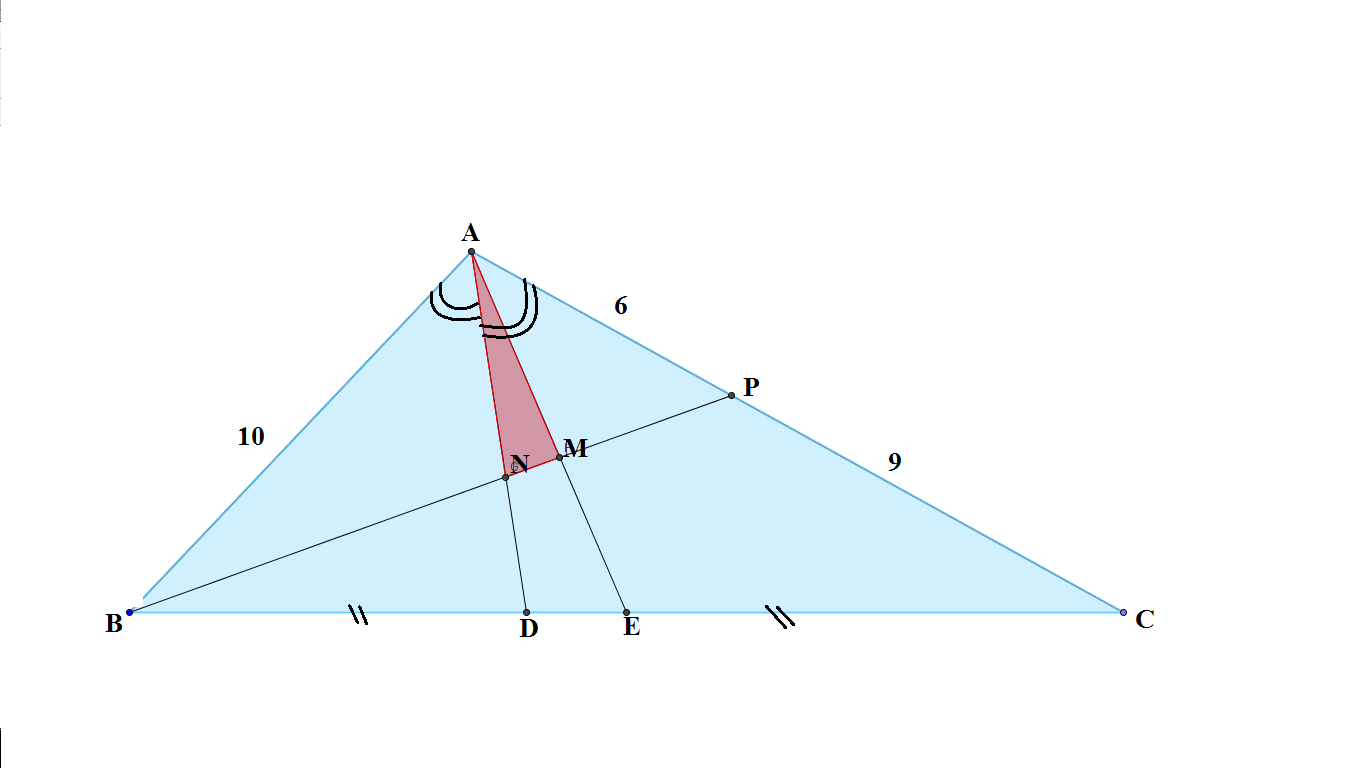# Triangle Formed By Intercepts

Geometry Level 4In $\triangle ABC$ $AB = 10$ and $AC = 15$. $AE$ is the median from $A$ and $AD$ is the internal bisector of $\angle BAC$. The point $P$ is on $AC$ such that $AP = 6$. $BP$ intersects $AD$ at $N$ and $AE$ at $M$. What is the value of

$\dfrac{[ABC]}{[ANM]}$ ?

×